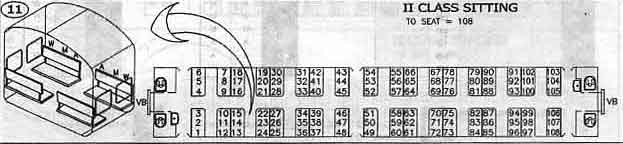# Seating Arrangement

## Problem:-

Akash and Vishal are quite fond of travelling. They mostly travel by railways. They were travelling in a train one day and they got interested in the seating arrangement of their compartment. The compartment looked something likeSo they got interested to know the seat number facing them and the seat type facing them. The seats are denoted as follows :

• Window Seat : WS
• Middle Seat : MS
• Aisle Seat : AS

You will be given a seat number, find out the seat number facing you and the seat type, i.e. WSMS or AS.

INPUT
First line of input will consist of a single integer T denoting number of test-cases. Each test-case consists of a single integer N denoting the seat-number.

OUTPUT
For each test case, print the facing seat-number and the seat-type, separated by a single space in a new line.

CONSTRAINTS

• 1<=T<=105
• 1<=N<=108
SAMPLE INPUT

`21840`
SAMPLE OUTPUT

`19 WS45 AS`
Time Limit:1.0 sec(s) for each input file.
Memory Limit:256 MB
Source Limit:1024 KB

## solution:-

#include<stdio.h>
void main()
{
long int t;
int n,i,j=1,r;
scanf(“%ld”,&t);
for(i=0;i<=t1;i++)
{
scanf(“%d”,&n);
while(j<13)
{
r=nj;
if(r%12==0)
break;
j++;
}
if(j==6)
{
printf(“%d “,n+1);
printf(“WSn”);
}
if(j==5)
{
printf(“%d “,n+3);
printf(“MSn”);
}
if(j==4)
{
printf(“%d “,n+5);
printf(“ASn”);
}
if(j==3)
{
printf(“%d “,n+7);
printf(“ASn”);
}
if(j==2)
{
printf(“%d “,n+9);
printf(“MSn”);
}
if(j==1)
{
printf(“%d “,n+11);
printf(“WSn”);
}
if(j==7)
{
printf(“%d “,n1);
printf(“WSn”);
}
if(j==8)
{
printf(“%d “,n3);
printf(“MSn”);
}
if(j==9)
{
printf(“%d “,n5);
printf(“ASn”);
}
if(j==10)
{
printf(“%d “,n7);
printf(“ASn”);
}
if(j==11)
{
printf(“%d “,n9);
printf(“MSn”);
}
if(j==12)
{
printf(“%d “,n11);
printf(“WSn”);
}
j=1;
}
}